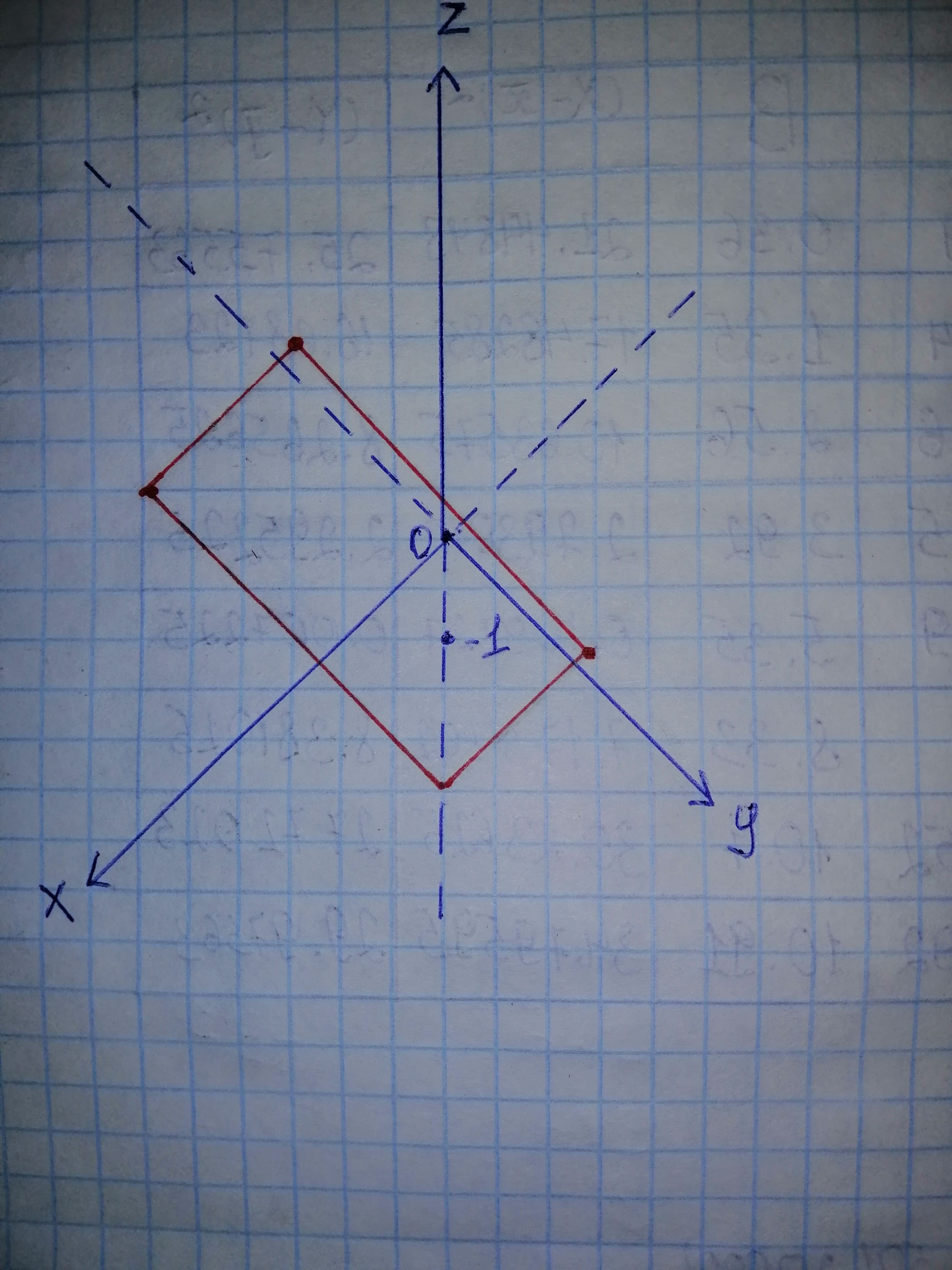# Describe in words the region of RR^{3} represented by the equation or inequality. z geq -1ka1leE 2020-11-23 Answered
Describe in words the region of $R{R}^{3}$ represented by the equation or inequality.
$z\ge -1$
You can still ask an expert for help

• Questions are typically answered in as fast as 30 minutes

Solve your problem for the price of one coffee

• Math expert for every subject
• Pay only if we can solve itBrighton

$R{R}^{3}$ is the three dimensional coordinate system which contains x, y, and z-coordinates.
The equation
represents the set $\left(x,y,z\right)|z\ge -1,$
which is the set of all points in $R{R}^{3}$ whose z-coordinate is greater than or equal to 8 and x, y-coordinates are any values.
The equation $z=-1$ is sketched as shown below in Figure 1.Thus, the equation
represents a half-space that consists of all points which are on or above the plane $z=-1.$